EcoDiet is a new tool for assimilating data in food-web studies. The goal of the package is to simultaneously estimate a probabilistic topology matrix and a diet matrix by combining biotracers and stomach content analyses in a Bayesian hierarchical model. The topology matrix contains all trophic link probabilities $$\eta$$, hence it gives for any prey the probability to be eaten by a given predator. The diet matrix contains all diet proportions $$\Pi$$, hence it gives for any prey the percentage of its contribution to a given predator's diet. The full model and its application on a real dataset are described in Hernvann et al. (under review). Use citation("EcoDiet") to get the full reference.

There are different ways of using this package:

• Default option - you have only stomach content and biotracers data, so non informative priors will be used;

• Literature option - you have stomach content and biotracers data, and results from a literature search that will be used to formulate informative priors.

The following example is an artificial dataset, created to be simple to visualize and understand. Note that the biotracers data used here are stable isotopes, but EcoDiet could be used to treat other analyses such as fatty acids or specific-compounds stable isotopes.

library(EcoDiet)

Your data should be in a specific format, similar to those of following example. You can load your data by importing it from .csv files or directly as R data.frames.

If you have the CSV files in a data folder and in a specific .csv format (semicolon separated, and not coma separated), you can try something like this:

example_stomach_data <- read.csv("./data/my_stomach_data.csv", sep = ";")
example_biotracer_data <- read.csv("./data/my_biotracer_data.csv", sep = ";")

Stomach content data

The stomach content table gathers the sum of occurrences of each prey species (or trophic group) in the stomachs of each trophic group. The first column of the table contains the names of the prey trophic group and the headers of the following columns contain the names of all the predator trophic groups. The last row of the table should be named “full”, and indicates how many (non-empty) stomachs have been analyzed for each trophic group.

example_stomach_data_path <- system.file("extdata", "example_stomach_data.csv",
package = "EcoDiet")
knitr::kable(example_stomach_data)
X huge large medium small
huge 0 0 0 0
large 11 0 0 0
medium 9 22 0 0
small 0 3 16 0
full 19 24 17 0

In this example, for the “huge” animals, 19 stomachs were analyzed and contained remainings. Among these stomachs, 17 contained “large” animal remainings and 12 contained “medium” animal remainings.

If you have trophic groups for which no stomach content analyses were done, you should fill the column with zeros (it is the case here for “small” animals that are at the base of the trophic network).

Biotracer data

Each line of the table represents one individual on which were conducted biotracer analyses for various elements (here stable isotope analyses for carbon and nitrogen). The first column of the table should be called “group” and indicates to which species or trophic group the individual belongs. The other columns contain the measures.

example_biotracer_data_path <- system.file("extdata", "example_biotracer_data.csv",
package = "EcoDiet")
knitr::kable(example_biotracer_data)
group d13C d15N
medium -15.64284 13.840550
medium -15.56745 12.600720
medium -16.44420 14.037290
medium -17.16711 13.133810
small -17.95860 10.443507
small -16.56196 10.789742
small -17.04855 11.236032
small -17.14060 8.976896
small -16.23605 8.580734
large -15.24835 16.433410
large -14.03405 16.299340
large -16.76164 17.009600
large -16.53439 15.878830
large -14.93301 16.633490
huge NA NA

You should have the same list of trophic groups in your stomach content and biotracer data. Thus, if you have a group with stomach content analyses but without biotracer data, you should still enter it as one line in the biotracer data with “NA” values (as it is the case for the “huge” group).

You also need to define the trophic discrimination factors corresponding to your biotracer data. In this example, we use the following trophic discrimination factors:

trophic_discrimination_factor = c(0.8, 3.4)

2. Preprocess your data without literature data (Default option)

If you have data extracted from the literature, skip this and go to section 3.

If you don't have literature data, read this section.

literature_configuration <- FALSE

Preprocess the data

The preprocess_data function checks and rearranges the data in a specific format so that the EcoDiet model can be run:

data <- preprocess_data(biotracer_data = example_biotracer_data,
trophic_discrimination_factor = c(0.8, 3.4),
literature_configuration = literature_configuration,
stomach_data = example_stomach_data)
#> The model will investigate the following trophic links:
#>        huge large medium small
#> huge      0     0      0     0
#> large     1     0      0     0
#> medium    1     1      0     0
#> small     0     1      1     0

If any error appears, it means your data is not in the correct format. Please read the error message and rearrange your data in the correct format.

If you have a lot of small values in the stomach occurences, you can choose to upscale the stomach content data:

data <- preprocess_data(biotracer_data = example_biotracer_data,
trophic_discrimination_factor = c(0.8, 3.4),
literature_configuration = literature_configuration,
stomach_data = example_stomach_data,
rescale_stomach = TRUE)
#> The model will investigate the following trophic links:
#>        huge large medium small
#> huge      0     0      0     0
#> large     1     0      0     0
#> medium    1     1      0     0
#> small     0     1      1     0

These are the links that will be investigated by the model. It is not wise to assume that all the trophic links are possible. You therefore need to consider only the reasonnable and important trophic links (e.g., a shrimp cannot eat a whale), as adding weak trophic links may introduce too much uncertainty in EcoDiet estimates. The matrix displayed by the preprocess_data function is based by default on the stomach content data (data$o): topology <- 1 * (data$o > 0)
print(topology)
#>        huge large medium small
#> huge      0     0      0     0
#> large     1     0      0     0
#> medium    1     1      0     0
#> small     0     1      1     0

If you want to add another trophic link, you can modify directly the binary topology matrix. It can be useful if your stomach sampling size is too low and you missed a prey you are definitely sure that the predator feeds on, or if the prey is little identifiable in stomachs (e.g., really small and highly digestible prey). To specify that the “huge” animal can also eat “small” animals, you can do:

topology["small", "huge"] <- 1
print(topology)
#>        huge large medium small
#> huge      0     0      0     0
#> large     1     0      0     0
#> medium    1     1      0     0
#> small     1     1      1     0

The new topology matrix can now be entered as an argument of the preprocess_data function:

data <- preprocess_data(biotracer_data = example_biotracer_data,
trophic_discrimination_factor = c(0.8, 3.4),
literature_configuration = literature_configuration,
topology = topology,
stomach_data = example_stomach_data)
#> The model will investigate the following trophic links:
#>        huge large medium small
#> huge      0     0      0     0
#> large     1     0      0     0
#> medium    1     1      0     0
#> small     1     1      1     0

3. Preprocess your data with literature data (Literature option)

If you don't have data extracted from the literature, skip this and go to section 4.

If you have literature data, read this section.

literature_configuration <- TRUE

Define the priors

A literature diet table is used to set priors on the trophic link probabilities $$\eta$$ and the diet proportions $$\Pi$$. This table is similar to the stomach contents table, as all trophic groups must be included in the columns and rows. The numbers are the average diet proportions found in the literature. Here, the selected studies have identified that “huge” animals eat equally “large” and “medium” animals (thus the 0.5 and 0.5 numbers in the first column). The proportions for a given predator (i.e., within a given column) must sum to 1. The “small” animals are at the base of the ecosystem, so the column is filled with zeros.

The last row of the table corresponds to the literature pedigree score. This score (a number from 0 to 1) quantifies the literature reliability on each predator's diet. Here the dietary proportions from the literature are used to produce reliable estimates for the “huge” animals, e.g., the pedigree score associated is high (0.9). On the contrary, the diet proportions for the “medium” animals come from an older article focusing on a very different ecosystem so estimates produced are less reliable, e.g, the pedigree score is low (0.2). The pedigree score for the “small” animals is set at 1, because this group eats nothing. For more details please read the reference article.

example_literature_diets_path <- system.file("extdata", "example_literature_diets.csv",
package = "EcoDiet")
knitr::kable(example_literature_diets)
X huge large medium small
huge 0.0 0.0 0.0 0
large 0.5 0.0 0.0 0
medium 0.5 0.7 0.0 0
small 0.0 0.3 1.0 0
pedigree 0.9 0.6 0.2 1

This summary of the literature data will be used to formulate:

1. The priors on the topology matrix's $\eta$s. If a given literature diet proportion is zero, the corresponding prior Beta distribution of $$\eta$$ will be shifted toward 0. If the proportion is positive, the distribution will be shifted toward 1.

2. The priors on the diet matrix's $\Pi$s. The literature diet proportions are entered as the hyperparameters of the prior Dirichlet distribution of $$\Pi$$.

The Pedigree scores are used to determine the priors' precision. Other parameters can be used to adjust the prior distributions:

• the nb_literature parameter. The higher the number, the stronger the weight will be of the literature in the final inference on $$\eta$$. Setting this parameter to 10 is like saying that the prior from the literature will weigh as much as the additional data from 10 stomachs. Thus for any particular application, nb_literature should be set to a value smaller than the sample size in the available stomach content data.
nb_literature = 10
• the literature_slope parameter (a value between 0 and 1). The higher the number, the stronger the weight will be of the literature in the final inference on $$\Pi$$. You should set this value depending on the value of your data (number of biotracers, etc).
literature_slope = 0.5

Preprocess the data

The preprocess_data function then checks and rearranges the data in a specific format so that the EcoDiet model can be run:

data <- preprocess_data(biotracer_data = example_biotracer_data,
trophic_discrimination_factor = c(0.8, 3.4),
literature_configuration = literature_configuration,
stomach_data = example_stomach_data,
literature_diets = example_literature_diets,
nb_literature = 10,
literature_slope = 0.5)
#> The model will investigate the following trophic links:
#>        huge large medium small
#> huge      0     0      0     0
#> large     1     0      0     0
#> medium    1     1      0     0
#> small     0     1      1     0

If any error appears, it means your data is not in the correct format. Please read the error message and try to rearrange the data in the correct format.

If you have a lot of small values in the stomach occurences, you can choose to upscale the stomach content data:

data <- preprocess_data(biotracer_data = example_biotracer_data,
trophic_discrimination_factor = c(0.8, 3.4),
literature_configuration = literature_configuration,
stomach_data = example_stomach_data,
rescale_stomach = TRUE,
literature_diets = example_literature_diets,
nb_literature = 10,
literature_slope = 0.5)
#> The model will investigate the following trophic links:
#>        huge large medium small
#> huge      0     0      0     0
#> large     1     0      0     0
#> medium    1     1      0     0
#> small     0     1      1     0

Check the trophic links to investigate

These are the links that will be investigated by the model. It is not wise to assume that all the trophic links are possible. You therefore need to keep only the reasonnable trophic links (e.g., a shrimp cannot eat a whale). The matrix displayed by the preprocess_data function is based by default on the stomach content data (data$o) and on the literature diet matrix (data$alpha_lit):

topology <- 1 * ((data$o > 0) | (data$alpha_lit > 0))
print(topology)
#>        huge large medium small
#> huge      0     0      0     0
#> large     1     0      0     0
#> medium    1     1      0     0
#> small     0     1      1     0

If you want to add another trophic link, you can modify directly the binary topology matrix. It can be useful if you are sure that a prey is consumed by a given predator. However the trophic link is not observed in the stomach content data, and the study extracted from the literature did not identify the prey. To specify that the “huge” animal can also eat “small” animals, you can do:

topology["small", "huge"] <- 1
print(topology)
#>        huge large medium small
#> huge      0     0      0     0
#> large     1     0      0     0
#> medium    1     1      0     0
#> small     1     1      1     0

The new topology matrix can now be entered as an argument of the preprocess_data function:

data <- preprocess_data(biotracer_data = example_biotracer_data,
trophic_discrimination_factor = c(0.8, 3.4),
literature_configuration = literature_configuration,
topology = topology,
stomach_data = example_stomach_data,
literature_diets = example_literature_diets,
nb_literature = 10,
literature_slope = 0.5)
#> The model will investigate the following trophic links:
#>        huge large medium small
#> huge      0     0      0     0
#> large     1     0      0     0
#> medium    1     1      0     0
#> small     1     1      1     0

4. Plot the data and the priors

You can visualize your data with the plot_data function:

plot_data(biotracer_data = example_biotracer_data,
stomach_data = example_stomach_data)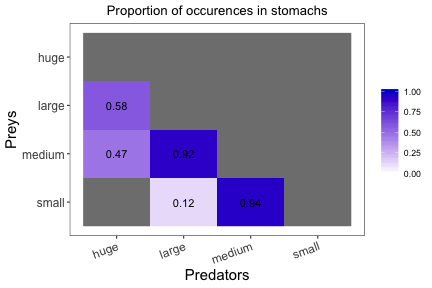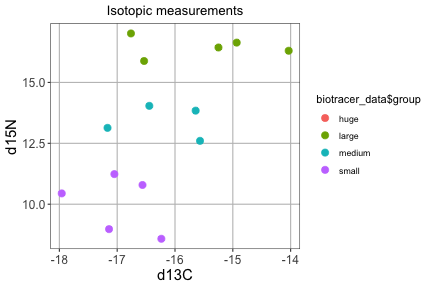You can save the figures as PNG in the current folder using:

plot_data(biotracer_data = example_biotracer_data,
stomach_data = example_stomach_data,
save = TRUE, save_path = ".")

Whether the priors are non-informative or informed by the literature, you can plot the mean of the prior distributions for the trophic link probabilities $$\eta$$ and the diet proportions $$\Pi$$:

plot_prior(data, literature_configuration)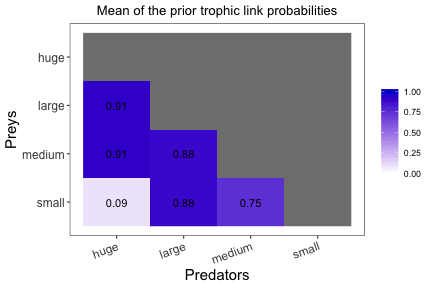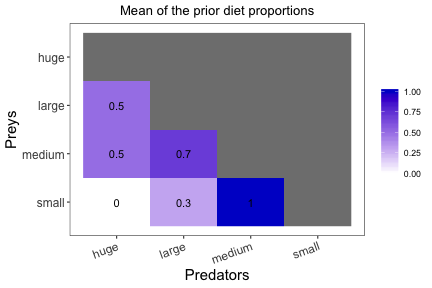You can also see the prior distributions for one trophic group (or predator):

plot_prior(data, literature_configuration, pred = "huge")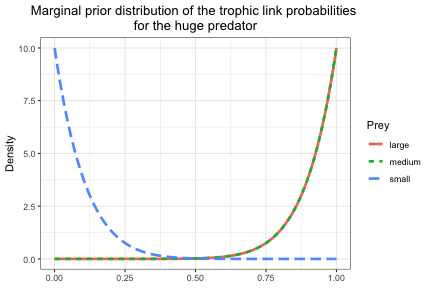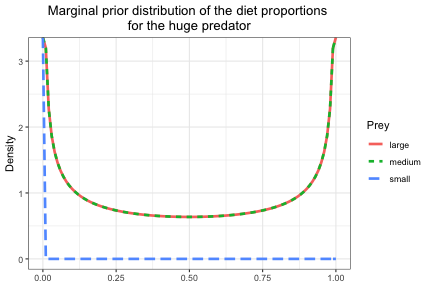This way, you can change the prior parameters and see how it affects the prior distributions. Here, we will change the nb_literature parameter from 10 to 2:

data <- preprocess_data(biotracer_data = example_biotracer_data,
trophic_discrimination_factor = c(0.8, 3.4),
literature_configuration = literature_configuration,
topology = topology,
stomach_data = example_stomach_data,
literature_diets = example_literature_diets,
nb_literature = 2,
literature_slope = 0.5)
#> The model will investigate the following trophic links:
#>        huge large medium small
#> huge      0     0      0     0
#> large     1     0      0     0
#> medium    1     1      0     0
#> small     1     1      1     0

plot_prior(data, literature_configuration, pred = "huge", variable = "eta")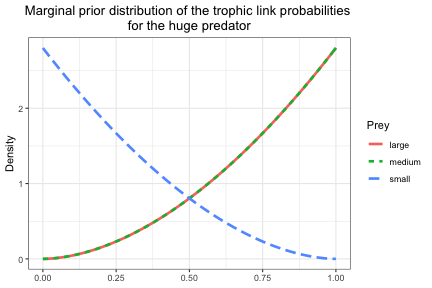5. Run the model

The write_model function writes the model in the BUGS syntax. You need to specify the option non informative priors / informative priors:

model_string <- write_model(literature_configuration = literature_configuration)

You can see the model with this command:

cat(model_string)

First run the model with low adaption and iteration numbers to test if it is compiling properly:

mcmc_output <- run_model(textConnection(model_string), data, nb_adapt = 1e1, nb_iter = 1e2)
#> Compiling model graph
#>    Resolving undeclared variables
#>    Allocating nodes
#> Graph information:
#>    Observed stochastic nodes: 20
#>    Unobserved stochastic nodes: 41
#>    Total graph size: 393
#>
#> Initializing model
#> Warning in rjags::jags.model(file = model_file, data = data, inits = inits, :
#> The model took 0.06 secs to be initialized.
#> The model took 0.19 secs to run.
#>
#>   /!\ CONVERGENCE PROBLEM /!\
#> Out of the 12 variables, 5 variables have a Gelman-Rubin statistic > 1.1.
#> You should increase the number of iterations of your model with the nb_iter argument.

The low numbers will surely not be enough to achieve a satisfactory model convergence. You should progressively increase the number of adaptation steps nb_adapt and of iterations nb_iter until you no longer see an “adaptation incomplete” warning, or a “convergence problem” message.

Depending on your data, the model can take hours or days to run:

mcmc_output <- run_model(textConnection(model_string), data, nb_adapt = 1e2, nb_iter = 1e3)
mcmc_output <- run_model(textConnection(model_string), data, nb_adapt = 1e3, nb_iter = 1e4)
mcmc_output <- run_model(textConnection(model_string), data, nb_adapt = 1e3, nb_iter = 1e5)
mcmc_output_example <- run_model(textConnection(model_string), data,
nb_adapt = 1e3, nb_iter = 1e6)

save(mcmc_output_example, file = "./data/mcmc_output_example.rda")

Don't forget to save the results before quitting R to not lose them.

6. Plot and save the results

The mean results

The model's outputs are the approximated a posteriori distributions for the trophic links probabilities $$\eta$$ and the diet proportions $$\Pi$$. You can visualize the mean of these distribitions with the plot_results function:

plot_results(mcmc_output_example, data)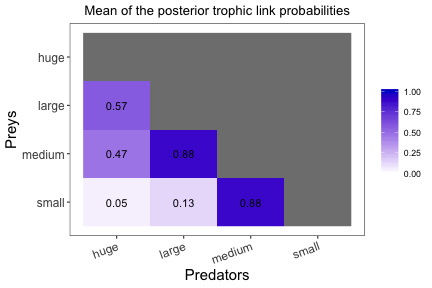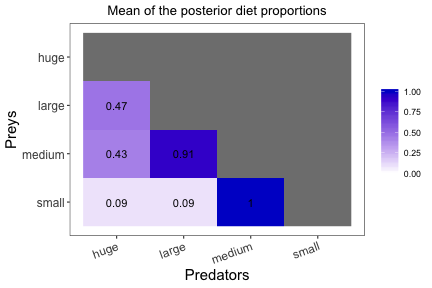You can access the mean value for each variable with the following:

print(colMeans(mcmc_output_example))
#>    PI[2,1]    PI[3,1]    PI[4,1]    PI[3,2]    PI[4,2]    PI[4,3]   eta[2,1]
#> 0.47324200 0.43315636 0.09360165 0.91401681 0.08598319 1.00000000 0.56630375
#>   eta[3,1]   eta[4,1]   eta[3,2]   eta[4,2]   eta[4,3]
#> 0.47036463 0.04786987 0.88273710 0.12721026 0.88410486

The probability distributions

The probability distributions can be plotted for one predator:

plot_results(mcmc_output_example, data, pred = "huge")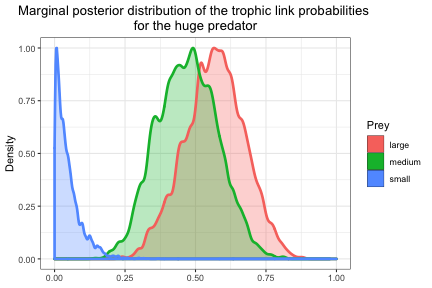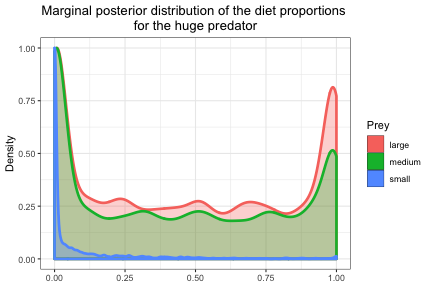plot_results(mcmc_output_example, data, pred = "large")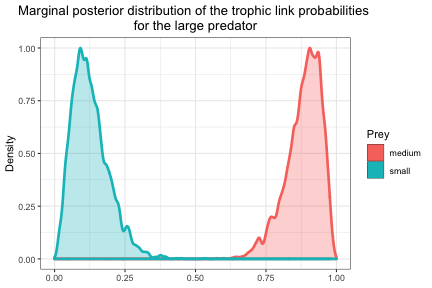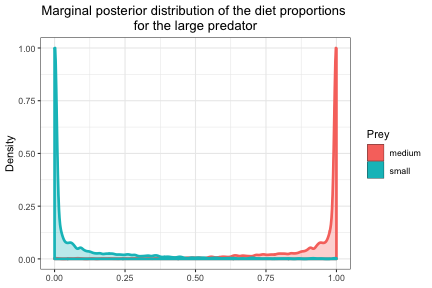As you can see, the shape of the posterior distributions of the $$\Pi$$ are unusual (with spikes at 0 and 1), and must be carefully interpreted in the model context. Indeed, $$\Pi$$ is conditionned by the trophic link existence $$\Lambda$$, a random Bernoulli variable taking the value 1 (the trophic link exists) or 0 (the trophic link does not exist):

• When $$\Lambda$$ = 0, $$\Pi$$ is drawn from a prior distribution with a spike at zero, with very low updating by the data.
• When $$\Lambda$$ = 1, $$\Pi$$ is updated by the data through the isotope mixture model.

The marginal distributions of $$\Pi$$ thus have a spike at zero combined with a more habitual dome shape centered on the value estimated through the mixture model. You can see the conditional distribution of $$\Pi$$ when the trophic link exist this way:

len <- dim(mcmc_output_example)
mcmc_output2 <- mcmc_output_example
mcmc_output2[, 1:(len/2)] <- ifelse(mcmc_output_example[, 1:(len/2)] < 0.03 |
mcmc_output_example[, 1:(len/2)] > 0.97,
NA, mcmc_output_example[, 1:(len/2)])

plot_results(mcmc_output2, data, variable = "PI", pred = "large", prey = c("medium", "small"))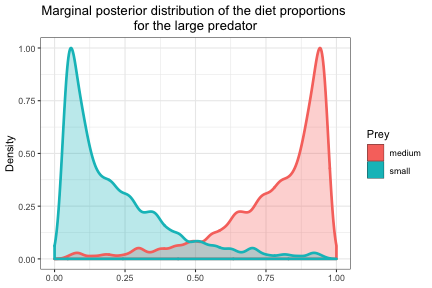You can also compute any summary statistics that you need. If you want the median (thus the 50% quantile), and the 5% and the 95% quantiles of your distribution, you can use:

quantiles <- apply(mcmc_output_example, 2, function(X) quantile(X, probs = c(0.05, 0.5, 0.95)))
quantiles <- signif(quantiles, digits = 2)
knitr::kable(quantiles)
PI[2,1] PI[3,1] PI[4,1] PI[3,2] PI[4,2] PI[4,3] eta[2,1] eta[3,1] eta[4,1] eta[3,2] eta[4,2] eta[4,3]
5% 1.0e-07 0.00 0.0000 0.58 0.0000 1 0.39 0.30 0.0031 0.76 0.039 0.74
50% 4.6e-01 0.39 0.0038 0.99 0.0064 1 0.57 0.47 0.0350 0.89 0.120 0.90
95% 1.0e+00 1.00 0.5100 1.00 0.4200 1 0.74 0.65 0.1400 0.96 0.250 0.98

7. Save another variable than $$\Pi$$ and $$\eta$$

You have the possibility to access all the model parameters. For example you may be interested by the variable $$\delta$$ that represents the trophic discrimination factor. In the EcoDiet model, a different trophic discrimination factor is used for each trophic group and for each element, allowing some differences between species. We can get these parameters using the variables_to_save argument:

mcmc_output <- run_model(textConnection(model_string), data,
variables_to_save = c("delta"),
nb_adapt = 1e1, nb_iter = 1e2)
#> Compiling model graph
#>    Resolving undeclared variables
#>    Allocating nodes
#> Graph information:
#>    Observed stochastic nodes: 20
#>    Unobserved stochastic nodes: 41
#>    Total graph size: 393
#>
#> Initializing model
#> Warning in rjags::jags.model(file = model_file, data = data, inits = inits, :
#> You should increase the number of iterations of your model with the nb_iter argument.